# Selina Solutions Concise Maths Class 10 Chapter 25 Probability Exercise 25(C)

This is the last exercise of the chapter and it contains a mixture of problems on finding probability. Students can refer to the Selina Solutions for Class 10 Maths when solving problems of this chapter and others as it contains very clear and detailed solutions to exercise problems. The solutions of this exercise are available in the Concise Selina Solutions for Class 10 Maths Chapter 25 Probability Exercise 25(C) PDF, which can be downloaded from the link below.

## Selina Solutions Concise Maths Class 10 Chapter 25 Probability Exercise 25(C) Download PDF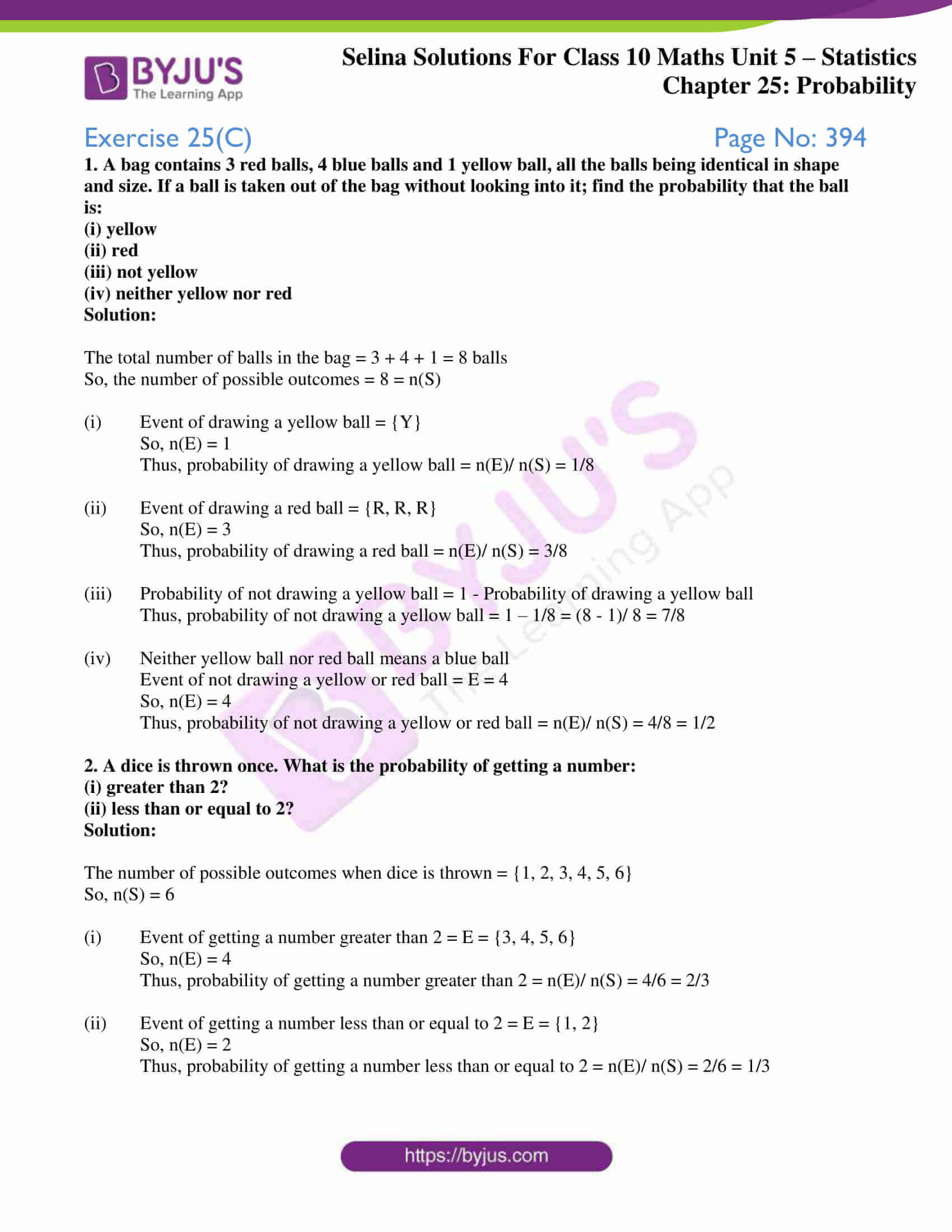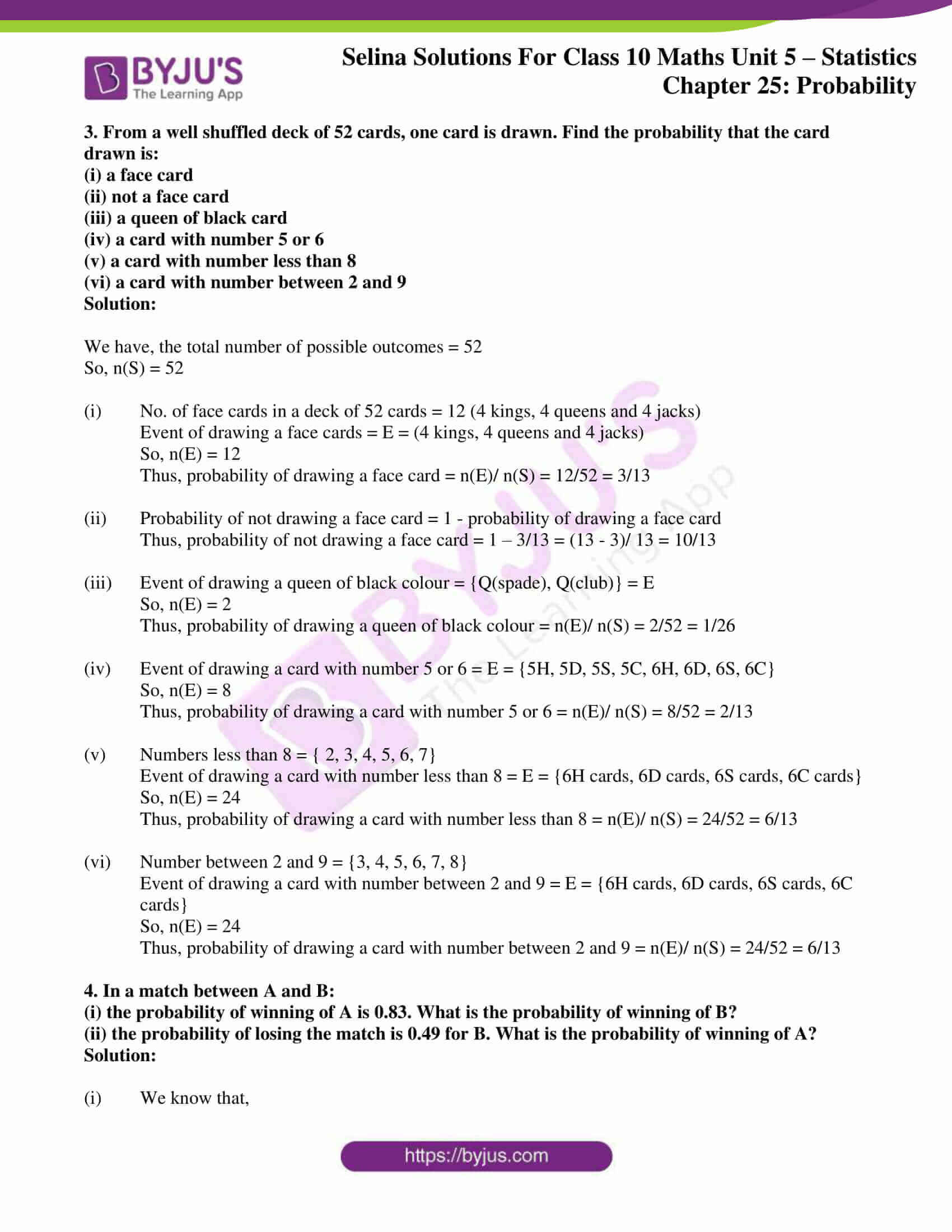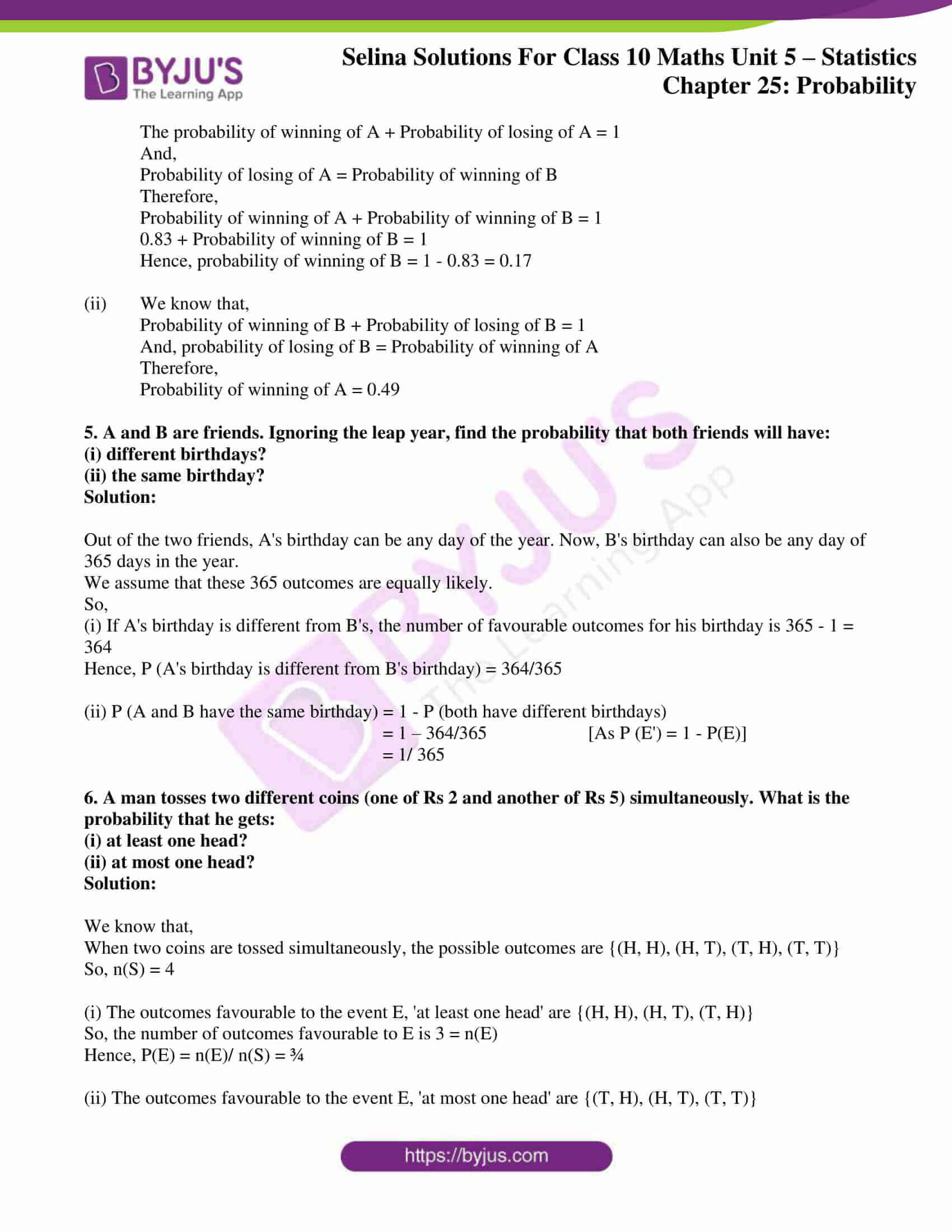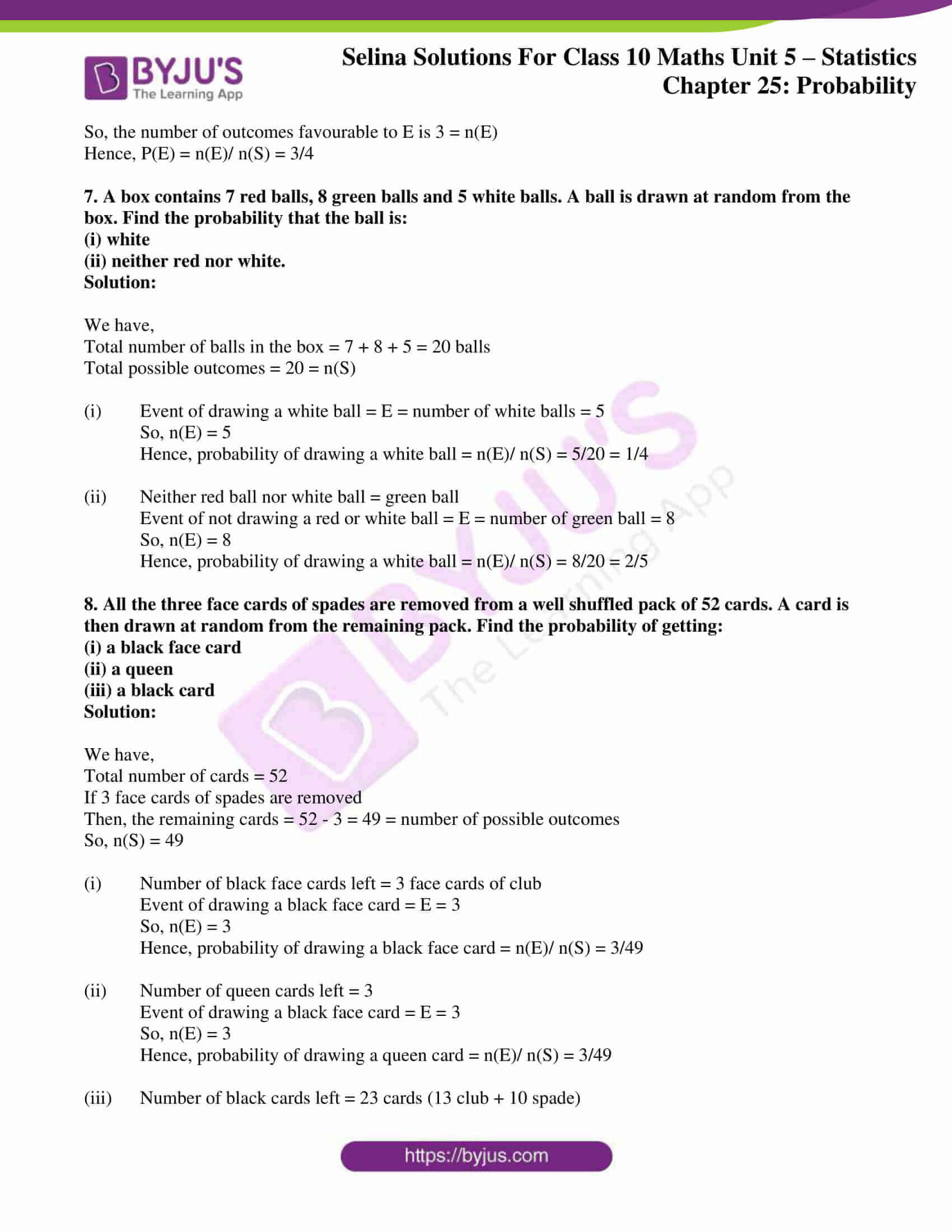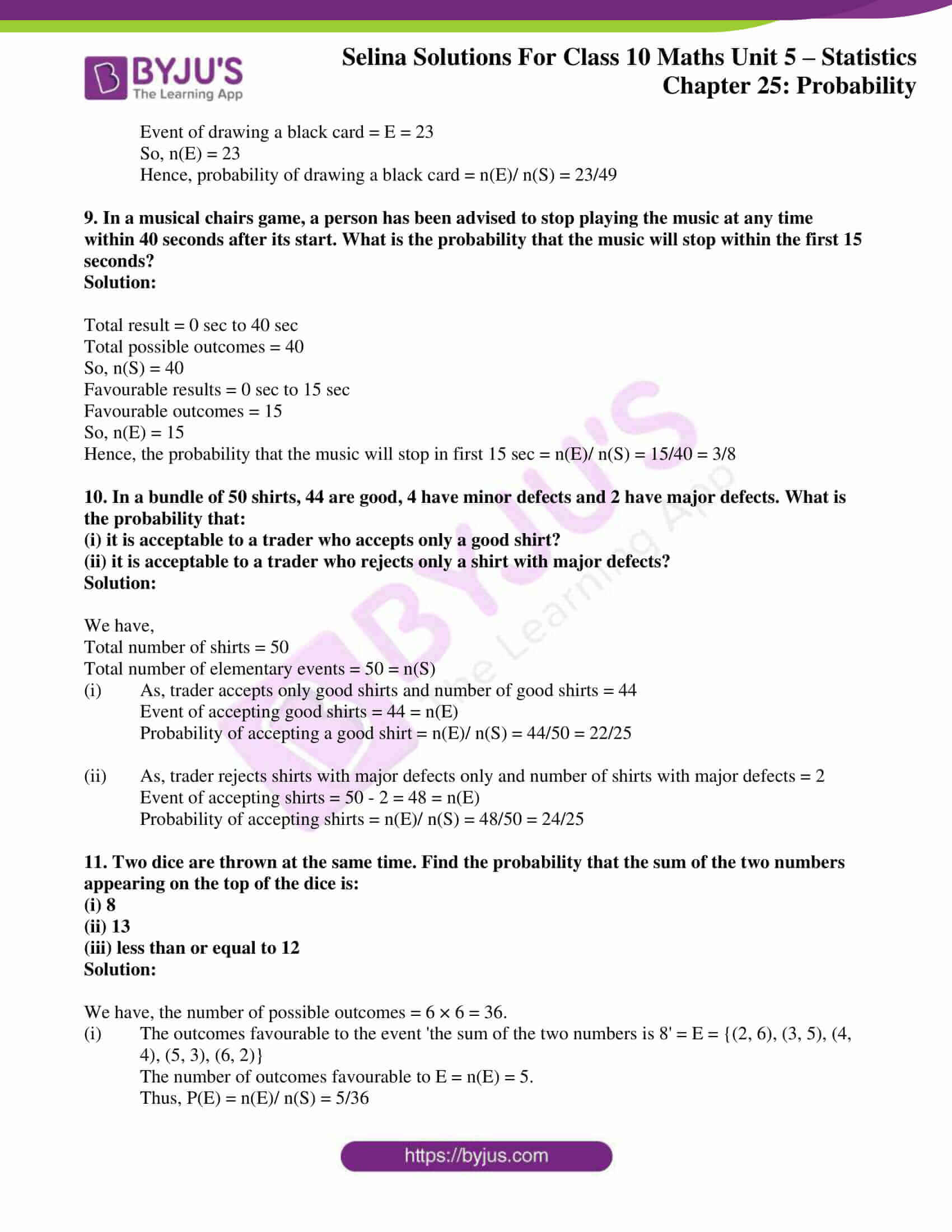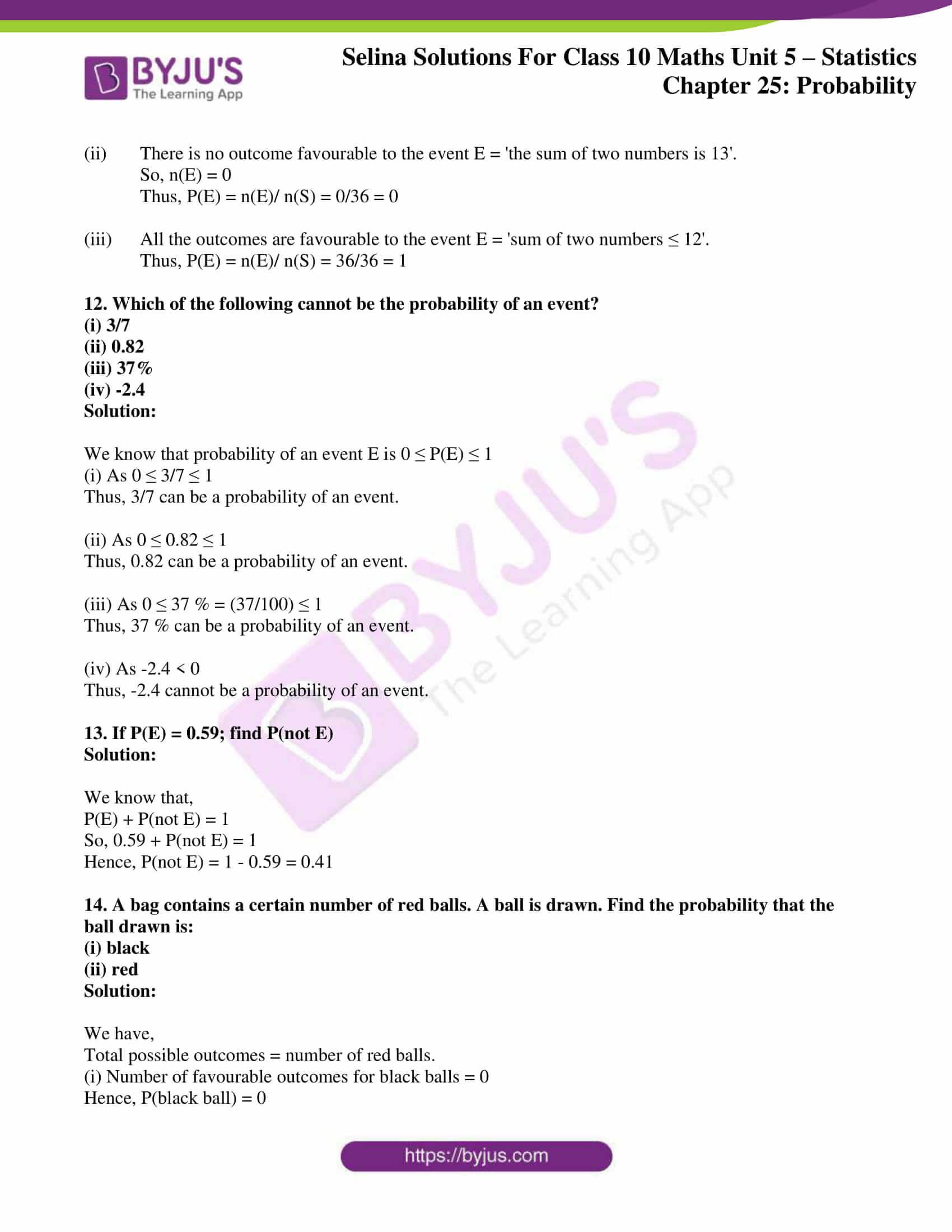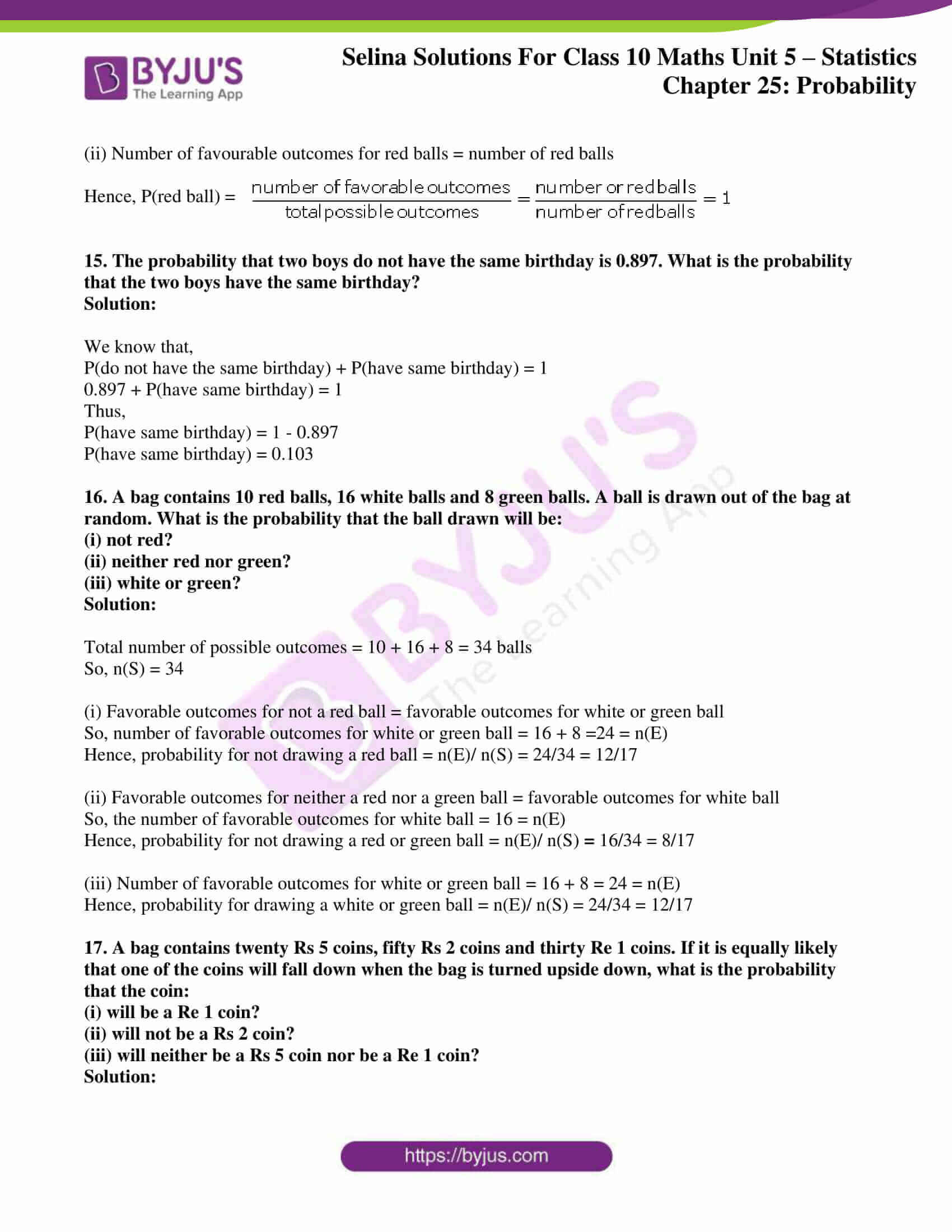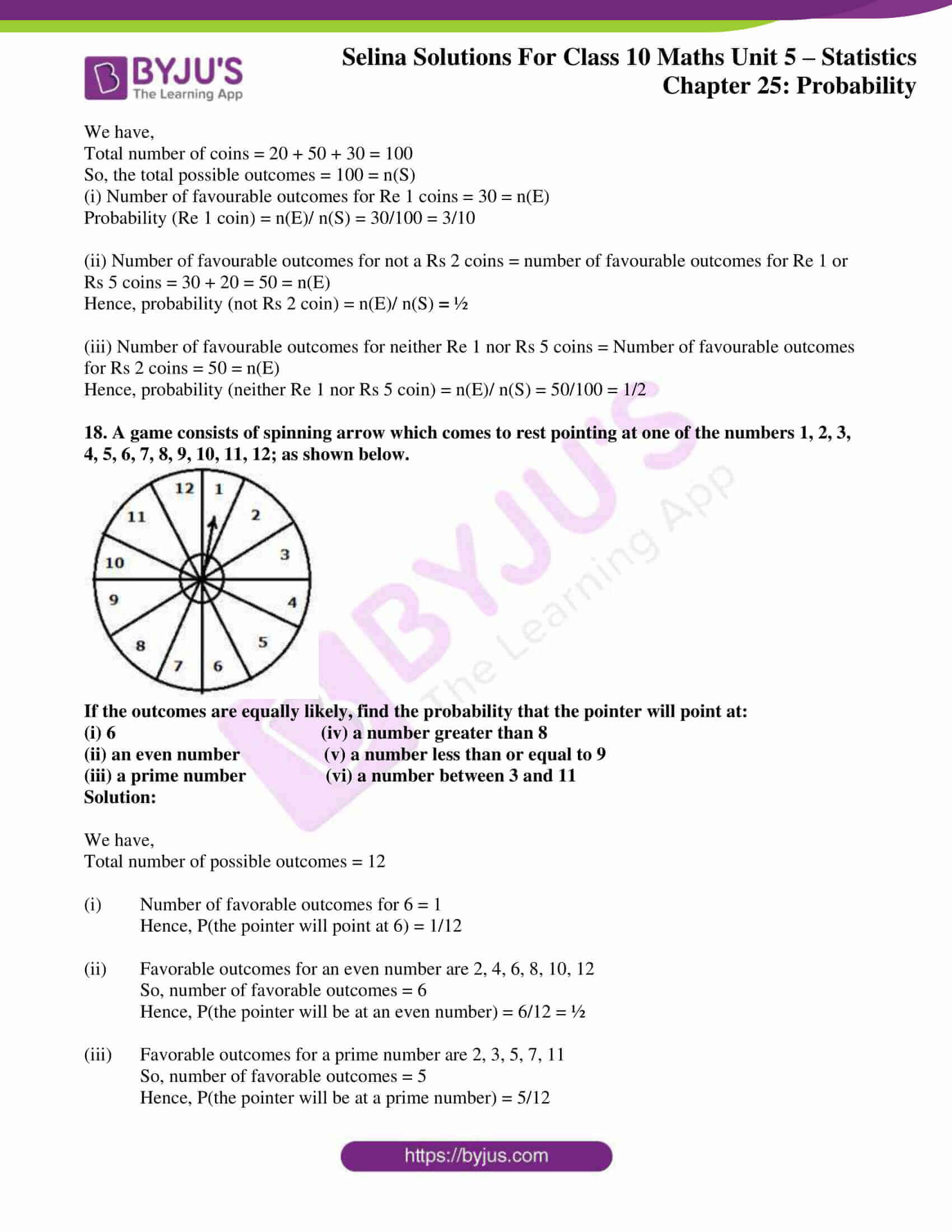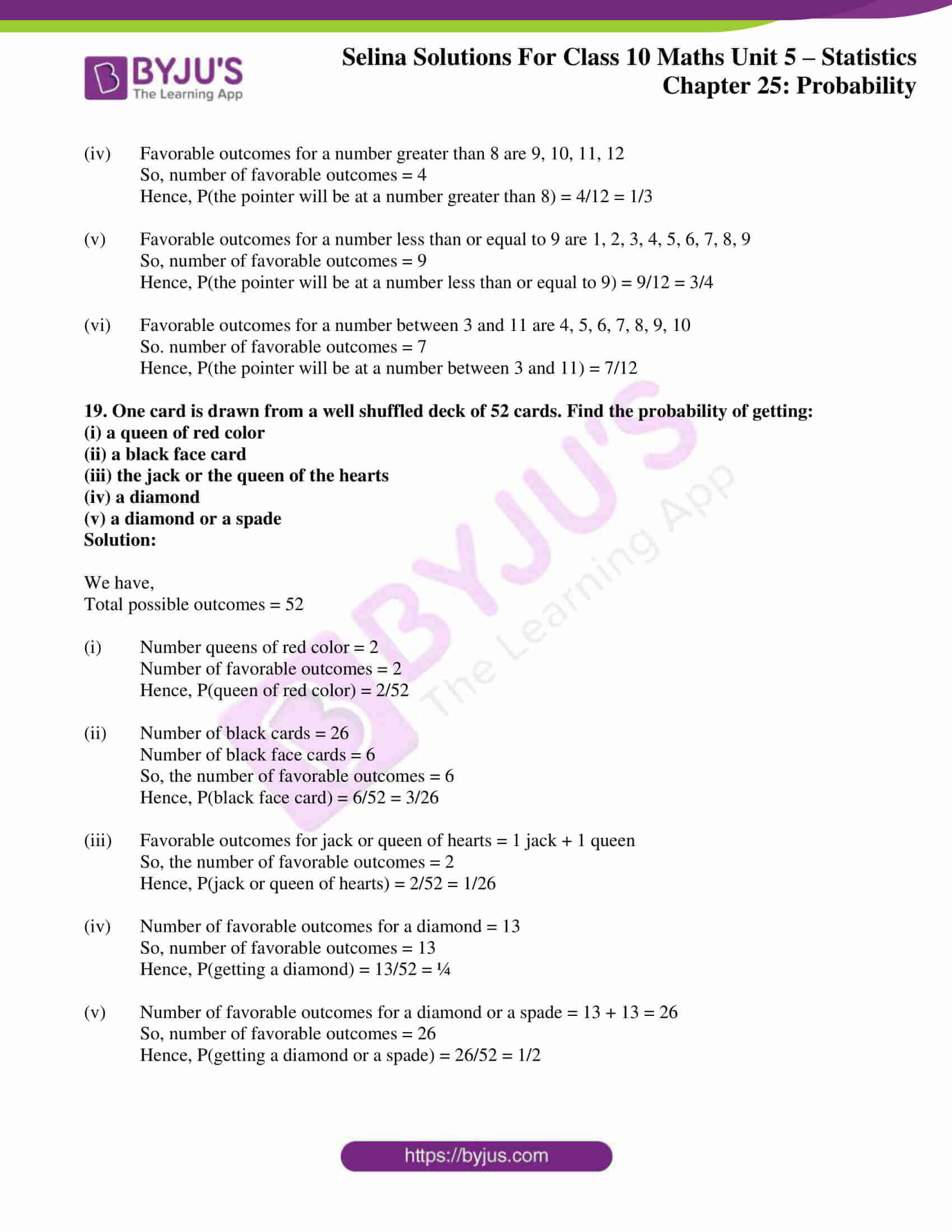### Access Selina Solutions Concise Maths Class 10 Chapter 25 Probability Exercise 25(C)

1. A bag contains 3 red balls, 4 blue balls and 1 yellow ball, all the balls being identical in shape and size. If a ball is taken out of the bag without looking into it; find the probability that the ball is:

(i) yellow

(ii) red

(iii) not yellow

(iv) neither yellow nor red

Solution:

The total number of balls in the bag = 3 + 4 + 1 = 8 balls

So, the number of possible outcomes = 8 = n(S)

(i) Event of drawing a yellow ball = {Y}

So, n(E) = 1

Thus, probability of drawing a yellow ball = n(E)/ n(S) = 1/8

(ii) Event of drawing a red ball = {R, R, R}

So, n(E) = 3

Thus, probability of drawing a red ball = n(E)/ n(S) = 3/8

(iii) Probability of not drawing a yellow ball = 1 – Probability of drawing a yellow ball

Thus, probability of not drawing a yellow ball = 1 – 1/8 = (8 – 1)/ 8 = 7/8

(iv) Neither yellow ball nor red ball means a blue ball

Event of not drawing a yellow or red ball = E = 4

So, n(E) = 4

Thus, probability of not drawing a yellow or red ball = n(E)/ n(S) = 4/8 = 1/2

2. A dice is thrown once. What is the probability of getting a number:

(i) greater than 2?

(ii) less than or equal to 2?

Solution:

The number of possible outcomes when dice is thrown = {1, 2, 3, 4, 5, 6}

So, n(S) = 6

(i) Event of getting a number greater than 2 = E = {3, 4, 5, 6}

So, n(E) = 4

Thus, probability of getting a number greater than 2 = n(E)/ n(S) = 4/6 = 2/3

(ii) Event of getting a number less than or equal to 2 = E = {1, 2}

So, n(E) = 2

Thus, probability of getting a number less than or equal to 2 = n(E)/ n(S) = 2/6 = 1/3

3. From a well shuffled deck of 52 cards, one card is drawn. Find the probability that the card drawn is:

(i) a face card

(ii) not a face card

(iii) a queen of black card

(iv) a card with number 5 or 6

(v) a card with number less than 8

(vi) a card with number between 2 and 9

Solution:

We have, the total number of possible outcomes = 52

So, n(S) = 52

(i)  No. of face cards in a deck of 52 cards = 12 (4 kings, 4 queens and 4 jacks)

Event of drawing a face cards = E = (4 kings, 4 queens and 4 jacks)

So, n(E) = 12

Thus, probability of drawing a face card = n(E)/ n(S) = 12/52 = 3/13

(ii) Probability of not drawing a face card = 1 – probability of drawing a face card

Thus, probability of not drawing a face card = 1 – 3/13 = (13 – 3)/ 13 = 10/13

(iii) Event of drawing a queen of black colour = {Q(spade), Q(club)} = E

So, n(E) = 2

Thus, probability of drawing a queen of black colour = n(E)/ n(S) = 2/52 = 1/26

(iv) Event of drawing a card with number 5 or 6 = E = {5H, 5D, 5S, 5C, 6H, 6D, 6S, 6C}

So, n(E) = 8

Thus, probability of drawing a card with number 5 or 6 = n(E)/ n(S) = 8/52 = 2/13

(v) Numbers less than 8 = { 2, 3, 4, 5, 6, 7}

Event of drawing a card with number less than 8 = E = {6H cards, 6D cards, 6S cards, 6C cards}

So, n(E) = 24

Thus, probability of drawing a card with number less than 8 = n(E)/ n(S) = 24/52 = 6/13

(vi) Number between 2 and 9 = {3, 4, 5, 6, 7, 8}

Event of drawing a card with number between 2 and 9 = E = {6H cards, 6D cards, 6S cards, 6C cards}

So, n(E) = 24

Thus, probability of drawing a card with number between 2 and 9 = n(E)/ n(S) = 24/52 = 6/13

4. In a match between A and B:

(i) the probability of winning of A is 0.83. What is the probability of winning of B?

(ii) the probability of losing the match is 0.49 for B. What is the probability of winning of A?

Solution:

(i) We know that,

The probability of winning of A + Probability of losing of A = 1

And,

Probability of losing of A = Probability of winning of B

Therefore,

Probability of winning of A + Probability of winning of B = 1

0.83 + Probability of winning of B = 1

Hence, probability of winning of B = 1 – 0.83 = 0.17

(ii) We know that,

Probability of winning of B + Probability of losing of B = 1

And, probability of losing of B = Probability of winning of A

Therefore,

Probability of winning of A = 0.49

5. A and B are friends. Ignoring the leap year, find the probability that both friends will have:

(i) different birthdays?

(ii) the same birthday?

Solution:

Out of the two friends, A’s birthday can be any day of the year. Now, B’s birthday can also be any day of 365 days in the year.

We assume that these 365 outcomes are equally likely.

So,

(i) If A’s birthday is different from B’s, the number of favourable outcomes for his birthday is 365 – 1 = 364

Hence, P (A’s birthday is different from B’s birthday) = 364/365

(ii) P (A and B have the same birthday) = 1 – P (both have different birthdays)

= 1 – 364/365 [As P (E’) = 1 – P(E)]

= 1/ 365

6. A man tosses two different coins (one of Rs 2 and another of Rs 5) simultaneously. What is the probability that he gets:

Solution:

We know that,

When two coins are tossed simultaneously, the possible outcomes are {(H, H), (H, T), (T, H), (T, T)}

So, n(S) = 4

(i) The outcomes favourable to the event E, ‘at least one head’ are {(H, H), (H, T), (T, H)}

So, the number of outcomes favourable to E is 3 = n(E)

Hence, P(E) = n(E)/ n(S) = ¾

(ii) The outcomes favourable to the event E, ‘at most one head’ are {(T, H), (H, T), (T, T)}

So, the number of outcomes favourable to E is 3 = n(E)

Hence, P(E) = n(E)/ n(S) = 3/4

7. A box contains 7 red balls, 8 green balls and 5 white balls. A ball is drawn at random from the box. Find the probability that the ball is:

(i) white

(ii) neither red nor white.

Solution:

We have,

Total number of balls in the box = 7 + 8 + 5 = 20 balls

Total possible outcomes = 20 = n(S)

(i) Event of drawing a white ball = E = number of white balls = 5

So, n(E) = 5

Hence, probability of drawing a white ball = n(E)/ n(S) = 5/20 = 1/4

(ii) Neither red ball nor white ball = green ball

Event of not drawing a red or white ball = E = number of green ball = 8

So, n(E) = 8

Hence, probability of drawing a white ball = n(E)/ n(S) = 8/20 = 2/5

8. All the three face cards of spades are removed from a well shuffled pack of 52 cards. A card is then drawn at random from the remaining pack. Find the probability of getting:

(i) a black face card

(ii) a queen

(iii) a black card

Solution:

We have,

Total number of cards = 52

If 3 face cards of spades are removed

Then, the remaining cards = 52 – 3 = 49 = number of possible outcomes

So, n(S) = 49

(i) Number of black face cards left = 3 face cards of club

Event of drawing a black face card = E = 3

So, n(E) = 3

Hence, probability of drawing a black face card = n(E)/ n(S) = 3/49

(ii) Number of queen cards left = 3

Event of drawing a black face card = E = 3

So, n(E) = 3

Hence, probability of drawing a queen card = n(E)/ n(S) = 3/49

(iii) Number of black cards left = 23 cards (13 club + 10 spade)

Event of drawing a black card = E = 23

So, n(E) = 23

Hence, probability of drawing a black card = n(E)/ n(S) = 23/49

9. In a musical chairs game, a person has been advised to stop playing the music at any time within 40 seconds after its start. What is the probability that the music will stop within the first 15 seconds?

Solution:

Total result = 0 sec to 40 sec

Total possible outcomes = 40

So, n(S) = 40

Favourable results = 0 sec to 15 sec

Favourable outcomes = 15

So, n(E) = 15

Hence, the probability that the music will stop in first 15 sec = n(E)/ n(S) = 15/40 = 3/8

10. In a bundle of 50 shirts, 44 are good, 4 have minor defects and 2 have major defects. What is the probability that:

(i) it is acceptable to a trader who accepts only a good shirt?

(ii) it is acceptable to a trader who rejects only a shirt with major defects?

Solution:

We have,

Total number of shirts = 50

Total number of elementary events = 50 = n(S)

(i) As, trader accepts only good shirts and number of good shirts = 44

Event of accepting good shirts = 44 = n(E)

Probability of accepting a good shirt = n(E)/ n(S) = 44/50 = 22/25

(ii) As, trader rejects shirts with major defects only and number of shirts with major defects = 2

Event of accepting shirts = 50 – 2 = 48 = n(E)

Probability of accepting shirts = n(E)/ n(S) = 48/50 = 24/25

11. Two dice are thrown at the same time. Find the probability that the sum of the two numbers appearing on the top of the dice is:

(i) 8

(ii) 13

(iii) less than or equal to 12

Solution:

We have, the number of possible outcomes = 6 × 6 = 36.

(i) The outcomes favourable to the event ‘the sum of the two numbers is 8’ = E = {(2, 6), (3, 5), (4, 4), (5, 3), (6, 2)}

The number of outcomes favourable to E = n(E) = 5.

Thus, P(E) = n(E)/ n(S) = 5/36

(ii) There is no outcome favourable to the event E = ‘the sum of two numbers is 13’.

So, n(E) = 0

Thus, P(E) = n(E)/ n(S) = 0/36 = 0

(iii) All the outcomes are favourable to the event E = ‘sum of two numbers ≤ 12’.

Thus, P(E) = n(E)/ n(S) = 36/36 = 1

12. Which of the following cannot be the probability of an event?

(i) 3/7

(ii) 0.82

(iii) 37%

(iv) -2.4

Solution:

We know that probability of an event E is 0 ≤ P(E) ≤ 1

(i) As 0 ≤ 3/7 ≤ 1

Thus, 3/7 can be a probability of an event.

(ii) As 0 ≤ 0.82 ≤ 1

Thus, 0.82 can be a probability of an event.

(iii) As 0 ≤ 37 % = (37/100) ≤ 1

Thus, 37 % can be a probability of an event.

(iv) As -2.4 < 0

Thus, -2.4 cannot be a probability of an event.

13. If P(E) = 0.59; find P(not E)

Solution:

We know that,

P(E) + P(not E) = 1

So, 0.59 + P(not E) = 1

Hence, P(not E) = 1 – 0.59 = 0.41

14. A bag contains a certain number of red balls. A ball is drawn. Find the probability that the ball drawn is:

(i) black

(ii) red

Solution:

We have,

Total possible outcomes = number of red balls.

(i) Number of favourable outcomes for black balls = 0

Hence, P(black ball) = 0

(ii) Number of favourable outcomes for red balls = number of red balls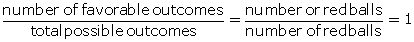Hence, P(red ball) =

15. The probability that two boys do not have the same birthday is 0.897. What is the probability that the two boys have the same birthday?

Solution:

We know that,

P(do not have the same birthday) + P(have same birthday) = 1

0.897 + P(have same birthday) = 1

Thus,

P(have same birthday) = 1 – 0.897

P(have same birthday) = 0.103

16. A bag contains 10 red balls, 16 white balls and 8 green balls. A ball is drawn out of the bag at random. What is the probability that the ball drawn will be:

(i) not red?

(ii) neither red nor green?

(iii) white or green?

Solution:

Total number of possible outcomes = 10 + 16 + 8 = 34 balls

So, n(S) = 34

(i) Favorable outcomes for not a red ball = favorable outcomes for white or green ball

So, number of favorable outcomes for white or green ball = 16 + 8 =24 = n(E)

Hence, probability for not drawing a red ball = n(E)/ n(S) = 24/34 = 12/17

(ii) Favorable outcomes for neither a red nor a green ball = favorable outcomes for white ball

So, the number of favorable outcomes for white ball = 16 = n(E)

Hence, probability for not drawing a red or green ball = n(E)/ n(S) = 16/34 = 8/17

(iii) Number of favorable outcomes for white or green ball = 16 + 8 = 24 = n(E)

Hence, probability for drawing a white or green ball = n(E)/ n(S) = 24/34 = 12/17

17. A bag contains twenty Rs 5 coins, fifty Rs 2 coins and thirty Re 1 coins. If it is equally likely that one of the coins will fall down when the bag is turned upside down, what is the probability that the coin:

(i) will be a Re 1 coin?

(ii) will not be a Rs 2 coin?

(iii) will neither be a Rs 5 coin nor be a Re 1 coin?

Solution:

We have,

Total number of coins = 20 + 50 + 30 = 100

So, the total possible outcomes = 100 = n(S)

(i) Number of favourable outcomes for Re 1 coins = 30 = n(E)

Probability (Re 1 coin) = n(E)/ n(S) = 30/100 = 3/10

(ii) Number of favourable outcomes for not a Rs 2 coins = number of favourable outcomes for Re 1 or Rs 5 coins = 30 + 20 = 50 = n(E)

Hence, probability (not Rs 2 coin) = n(E)/ n(S) = ½

(iii) Number of favourable outcomes for neither Re 1 nor Rs 5 coins = Number of favourable outcomes for Rs 2 coins = 50 = n(E)

Hence, probability (neither Re 1 nor Rs 5 coin) = n(E)/ n(S) = 50/100 = 1/2

18. A game consists of spinning arrow which comes to rest pointing at one of the numbers 1, 2, 3, 4, 5, 6, 7, 8, 9, 10, 11, 12; as shown below.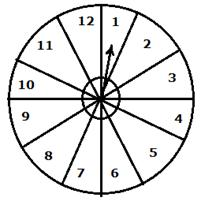If the outcomes are equally likely, find the probability that the pointer will point at:

(i) 6                                            (iv) a number greater than 8

(ii) an even number                  (v) a number less than or equal to 9

(iii) a prime number                 (vi) a number between 3 and 11

Solution:

We have,

Total number of possible outcomes = 12

(i) Number of favorable outcomes for 6 = 1

Hence, P(the pointer will point at 6) = 1/12

(ii) Favorable outcomes for an even number are 2, 4, 6, 8, 10, 12

So, number of favorable outcomes = 6

Hence, P(the pointer will be at an even number) = 6/12 = ½

(iii) Favorable outcomes for a prime number are 2, 3, 5, 7, 11

So, number of favorable outcomes = 5

Hence, P(the pointer will be at a prime number) = 5/12

(iv) Favorable outcomes for a number greater than 8 are 9, 10, 11, 12

So, number of favorable outcomes = 4

Hence, P(the pointer will be at a number greater than 8) = 4/12 = 1/3

(v) Favorable outcomes for a number less than or equal to 9 are 1, 2, 3, 4, 5, 6, 7, 8, 9

So, number of favorable outcomes = 9

Hence, P(the pointer will be at a number less than or equal to 9) = 9/12 = 3/4

(vi) Favorable outcomes for a number between 3 and 11 are 4, 5, 6, 7, 8, 9, 10

So. number of favorable outcomes = 7

Hence, P(the pointer will be at a number between 3 and 11) = 7/12

19. One card is drawn from a well shuffled deck of 52 cards. Find the probability of getting:

(i) a queen of red color

(ii) a black face card

(iii) the jack or the queen of the hearts

(iv) a diamond

(v) a diamond or a spade

Solution:

We have,

Total possible outcomes = 52

(i) Number queens of red color = 2

Number of favorable outcomes = 2

Hence, P(queen of red color) = 2/52

(ii) Number of black cards = 26

Number of black face cards = 6

So, the number of favorable outcomes = 6

Hence, P(black face card) = 6/52 = 3/26

(iii) Favorable outcomes for jack or queen of hearts = 1 jack + 1 queen

So, the number of favorable outcomes = 2

Hence, P(jack or queen of hearts) = 2/52 = 1/26

(iv) Number of favorable outcomes for a diamond = 13

So, number of favorable outcomes = 13

Hence, P(getting a diamond) = 13/52 = ¼

(v) Number of favorable outcomes for a diamond or a spade = 13 + 13 = 26

So, number of favorable outcomes = 26

Hence, P(getting a diamond or a spade) = 26/52 = 1/2